## 11.1.3 Defining a Planning Problem

Planning problems will be defined directly on the history I-space, which makes it appear as an ordinary state space in many ways. Keep in mind, however, that it was derived from another state space for which perfect state observations could not be obtained. In Section 10.1, a feedback plan was defined as a function of the state. Here, a feedback plan is instead a function of the I-state. Decisions cannot be based on the state because it will be generally unknown during the execution of the plan. However, the I-state is always known; thus, it is logical to base decisions on it.

Let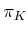denote a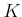-step information-feedback plan, which is a sequence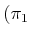,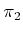,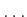,) offunctions,. Thus, at every stage, the I-state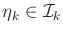is used as a basis for choosing the action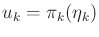. Due to interference of nature through both the state transition equation and the sensor mapping, the action sequence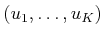produced by a plan,, will not be known until the plan terminates.

As in Formulation 2.3, it will be convenient to assume that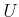contains a termination action,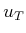. Ifis applied at stage, then it is repeatedly applied forever. It is assumed once again that the state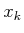remains fixed after the termination condition is applied. Remember, however,is still unknown in general; it becomes fixed but unknown. Technically, based on the definition of the history I-space, the I-state must change afteris applied because the history grows. These changes can be ignored, however, because no new decisions are made afteris applied. A plan that uses a termination condition can be specified as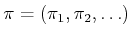because the number of stages may vary each time the plan is executed. Using the history I-space definition in (11.19), an information-feedback plan is expressed as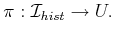(11.20)

We are almost ready to define the planning problem. This will require the specification of a cost functional. The cost depends on the histories of states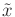and actions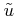as in Section 10.1. The planning formulation involves the following components, summarizing most of the concepts introduced so far in Section 11.1 (see Formulation 10.1 for similarities):

Formulation 11..1 (Discrete Information Space Planning)
1. A nonempty state spacethat is either finite or countably infinite.
2. A nonempty, finite action space. It is assumed thatcontains a special termination action, which has the same effect as defined in Formulation 2.3.
3. A finite nature action space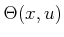for each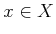and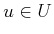.
4. A state transition function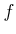that produces a state,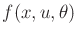, for every,, and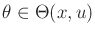.
5. A finite or countably infinite observation space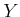.
6. A finite nature sensing action space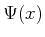for each.
7. A sensor mapping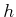which produces an observation,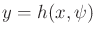, for eachand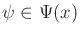. This definition assumes a state-nature sensor mappings. A state sensor mapping or history-based sensor mapping, as defined in Section 11.1.1, could alternatively be used.
8. A set of stages, each denoted by, which begins at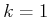and continues indefinitely.
9. An initial condition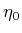, which is an element of an initial condition space,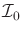.
10. A history I-space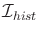which is the union ofand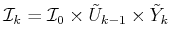for every stage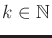.
11. Let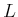denote a stage-additive cost functional, which may be applied to any pair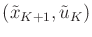of state and action histories to yield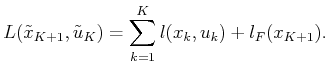(11.21)

If the termination actionis applied at some stage, then for all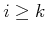,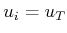,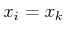, and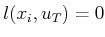. Either a feasible or optimal planning problem can be defined, as in Formulation 10.1; however, the plan here is specified as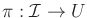.

A goal set may be defined as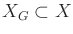. Alternatively, the goal could be expressed as a desirable set of history I-states. After Section 11.2, it will be seen that the goal can be expressed in terms of I-states that are derived from histories.

Some immediate extensions of Formulation 11.1 are possible, but we avoid them here simplify notation in the coming concepts. One extension is to allow different action sets,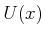, for each. Be careful, however, because information regarding the current state can be inferred if the action setis given, and it varies depending on. Another extension is to allow the costs to depend on nature, to obtain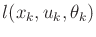, instead of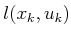in (11.21).

Subsections
Steven M LaValle 2020-08-14